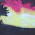# Programming Videos

## Search

### C Program to Calculate the Sum of First N Natural Numbers

Write a C program to calculate the sum of first n natural numbers. Given an input n, We have to write a code to print the sum of first n natural number (1 to n).

There are multiple approaches to solve this problem.

* We can solve this problem using loop.
* Other approach is to use mathematical formula to calculate the sum of first n natural number.
* We can also use recursion to find the sum of first n natural numbers using recursion.

Suppose if user has entered a number 10. So, we have to calculate the sum of first 10 numbers ( 1 to 10). The Sum of first 10 numbers is 55.

Program to Calculate Sum of Digits of a Number

### How to Calculate the Sum of first N Natural Numbers

1. First approach is to use for loop to calculate the sum of first n natural numbers.

```  for(i=1; i<=num; i++){

sum +=i;
}
```

Program to Count Number of Words in a Sentence by Taking Input from User

2. Another approach is to use mathematical formula n(n+1)/2 to calculate the sum of first n natural numbers.

Suppose, If user has entered the value of n is 20 = 20 * (20 + 1)/2 = 210

The sum of first 20 numbers is 210.

C, C++ Interview Questions.

## C Program to Calculate the Sum of First n Natural Numbers using Mathematical Formula

We have discussed multiple approaches to calculate the sum of first n natural numbers. Let's write a code to solve this problem using discussed approaches.

In this approach, we are calculating the sum of first n natural numbers using mathematical formula.

```#include <stdio.h>

int main()
{

int n,sum;

printf("Enter the value of n");
scanf("%d",&n);

sum = (n*(n+1))/2;

printf("The sum of n number is %d",sum);

return 0;

}
```

## C Program to Calculate Sum of First n Natural Numbers using For Loop

```#include <stdio.h>

int main(void) {

int n,sum=0,i;

printf("Enter the value of n");
scanf("%d",&n);

/* Iterate from 1 to n . */

for(i=1; i<=n; i++) {

sum = sum + i;
}

printf("The sum of n number is %d",sum);

return 0;
}```

Find the Sum of First N Odd Numbers

Output:

Enter the value of n : 10

The sum of n number is : 55

Programming questions on Strings

Programming questions on Recursion

Programming questions on Array

1.